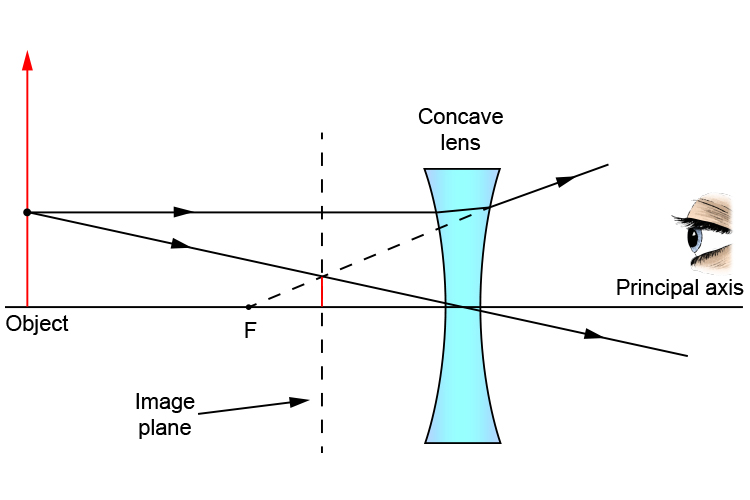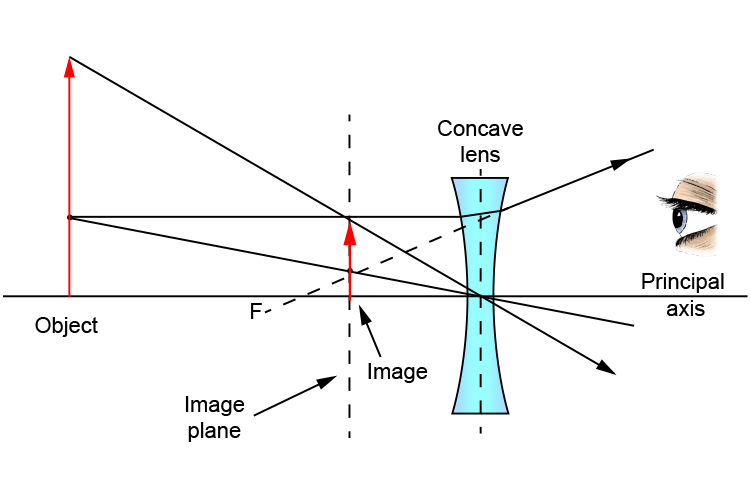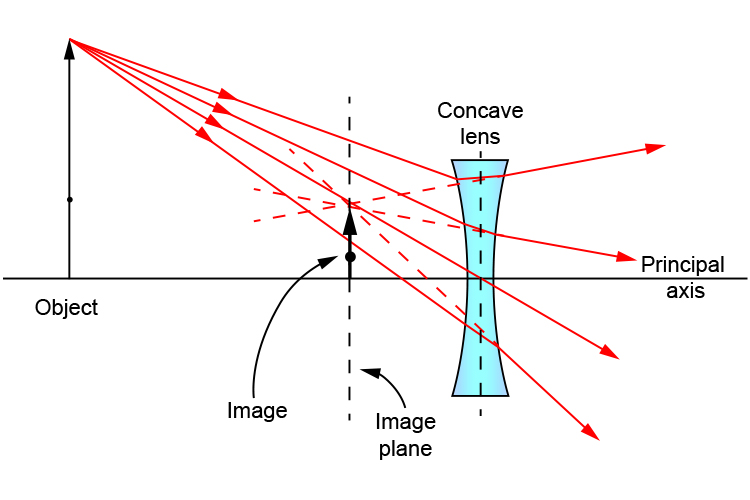# Concave lens and objects larger than the lens

So far all our objects have been smaller than the concave lens. What happens when the object is larger than the concave lens?

Locate one point on the object that applies to the ray diagram rules and this will give you your image plane.

Follows these simple stages:Take two lines from the object. Remember:

1. Pass a parallel line through the principal focal point on the same side as the object.
2. Pass a ray line through the centre of the concave lens.

Now you have the image plane. Draw a line anywhere on the object through the centre of the concave lens and wherever it passes the image plane is the point of focus.Now you know where the image is placed, any other rays can be predicted as follows: